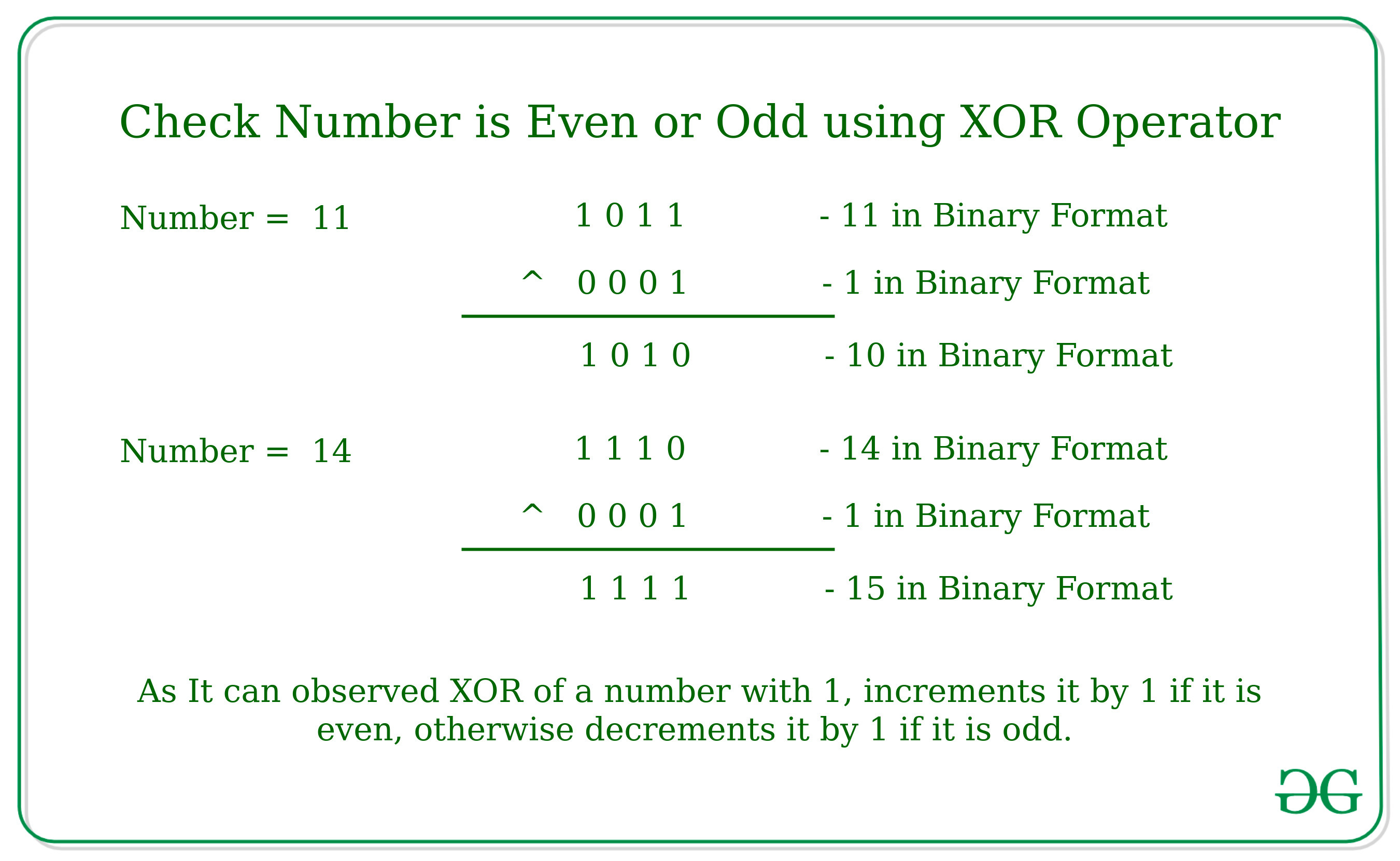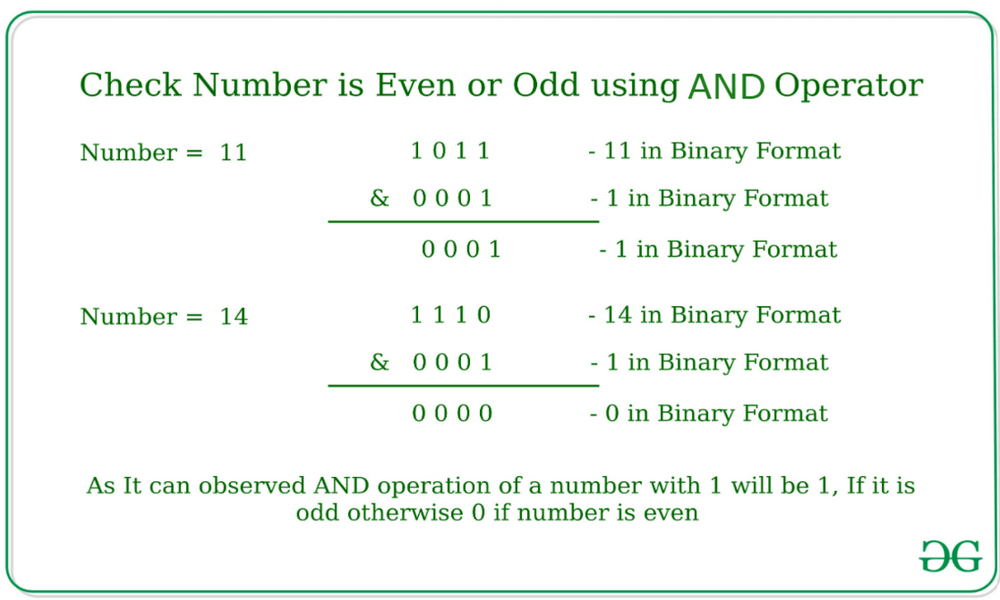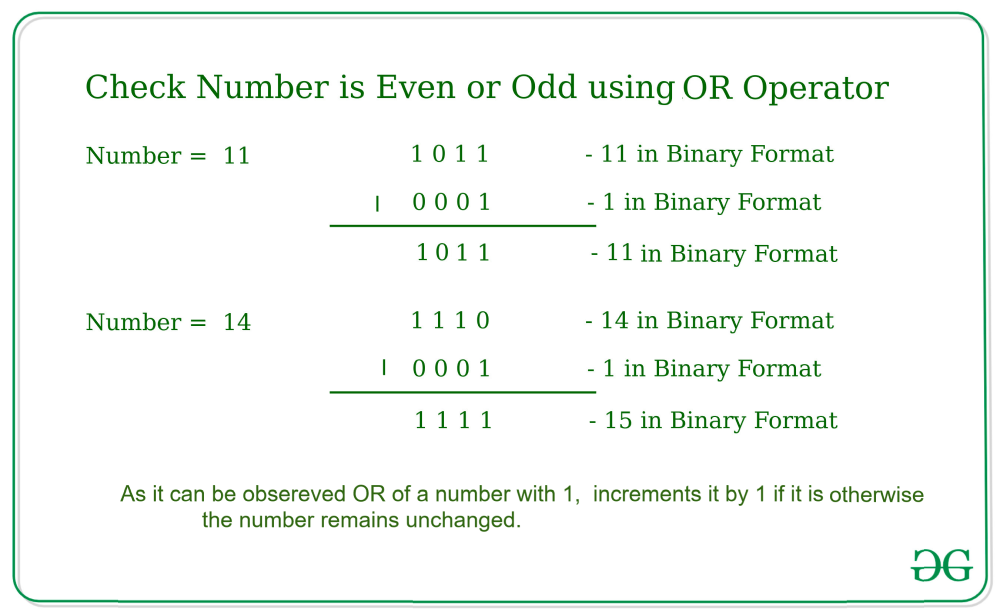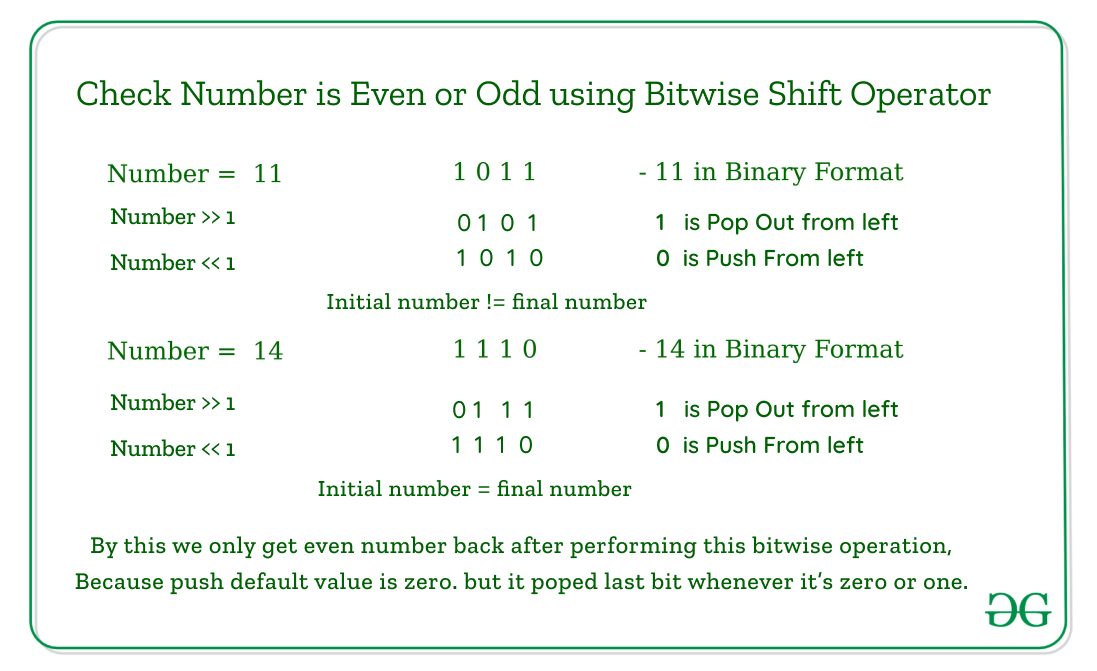Open in App
Not now

# Check if a Number is Odd or Even using Bitwise Operators

• Difficulty Level : Basic
• Last Updated : 01 Feb, 2023

Given a number N, the task is to check whether the number is even or odd using Bitwise Operators.

Examples:

Input: N = 11
Output: Odd

Input: N = 10
Output: Even

Following Bitwise Operators can be used to check if a number is odd or even:

1. Using Bitwise XOR operator:
The idea is to check whether the last bit of the number is set or not. If the last bit is set then the number is odd, otherwise even.
As we know bitwise XOR Operation of the Number by 1 increment the value of the number by 1 if the number is even otherwise it decrements the value of the number by 1 if the value is odd.Below is the implementation of the above approach:

## C++

 `// C++ program to check for even or odd``// using Bitwise XOR operator` `#include ``using` `namespace` `std;` `// Returns true if n is even, else odd``bool` `isEven(``int` `n)``{` `    ``// n^1 is n+1, then even, else odd``    ``if` `((n ^ 1) == (n + 1))``        ``return` `true``;``    ``else``        ``return` `false``;``}` `// Driver code``int` `main()``{``    ``int` `n = 100;``    ``isEven(n) ? cout << ``"Even"` `: cout << ``"Odd"``;` `    ``return` `0;``}`

## Java

 `// Java program to check for even or odd``// using Bitwise XOR operator``class` `GFG {` `    ``// Returns true if n is even, else odd``    ``static` `boolean` `isEven(``int` `n)``    ``{` `        ``// n^1 is n+1, then even, else odd``        ``if` `((n ^ ``1``) == (n + ``1``))``            ``return` `true``;``        ``else``            ``return` `false``;``    ``}` `    ``// Driver code``    ``public` `static` `void` `main(String[] args)``    ``{``        ``int` `n = ``100``;``        ``System.out.print(isEven(n) == ``true` `? ``"Even"``                                           ``: ``"Odd"``);``    ``}``}` `// This code is contributed by Rajput-Ji`

## Python3

 `# Python3 program to check for even or odd``# using Bitwise XOR operator` `# Returns true if n is even, else odd`  `def` `isEven(n):` `    ``# n^1 is n+1, then even, else odd``    ``if` `((n ^ ``1``) ``=``=` `(n ``+` `1``)):``        ``return` `True``    ``else``:``        ``return` `False`  `# Driver code``if` `__name__ ``=``=` `"__main__"``:``    ``n ``=` `100``    ``print``(``"Even"``) ``if` `isEven(n) ``else` `print``(``"Odd"``)` `# This code is contributed by AnkitRai01`

## C#

 `// C# program to check for even or odd``// using Bitwise XOR operator``using` `System;` `class` `GFG {` `    ``// Returns true if n is even, else odd``    ``static` `bool` `isEven(``int` `n)``    ``{` `        ``// n^1 is n+1, then even, else odd``        ``if` `((n ^ 1) == (n + 1))``            ``return` `true``;``        ``else``            ``return` `false``;``    ``}` `    ``// Driver code``    ``public` `static` `void` `Main(String[] args)``    ``{``        ``int` `n = 100;``        ``Console.Write(isEven(n) == ``true` `? ``"Even"` `: ``"Odd"``);``    ``}``}` `// This code is contributed by Rajput-Ji`

## Javascript

 ``

Output

`Even`

Time Complexity: O(1)
Auxiliary Space: O(1)

2. Using Bitwise AND operator:
The idea is to check whether the last bit of the number is set or not. If last bit is set then the number is odd, otherwise even.
As we know bitwise AND Operation of the Number by 1 will be 1, If it is odd because the last bit will be already set. Otherwise, it will give 0 as output.Below is the implementation of the above approach:

## C++

 `// C++ program to check for even or odd``// using Bitwise AND operator``#include ``using` `namespace` `std;` `// Returns true if n is even, else odd``bool` `isEven(``int` `n)``{``    ``// n&1 is 1, then odd, else even``    ``return` `(!(n & 1));``}` `// Driver code``int` `main()``{``    ``int` `n = 101;``    ``isEven(n) ? cout << ``"Even"` `: cout << ``"Odd"``;``    ``return` `0;``}`

## Java

 `// Java program to check for even or odd``// using Bitwise AND operator``class` `GFG {` `    ``// Returns true if n is even, else odd``    ``static` `boolean` `isEven(``int` `n)``    ``{``        ``// n&1 is 1, then odd, else even``        ``return` `((n & ``1``) != ``1``);``    ``}` `    ``// Driver code``    ``public` `static` `void` `main(String[] args)``    ``{``        ``int` `n = ``101``;``        ``System.out.print(isEven(n) == ``true` `? ``"Even"``                                           ``: ``"Odd"``);``    ``}``}` `// This code is contributed by PrinciRaj1992`

## Python3

 `# Python3 program to check for even or odd``# using Bitwise AND operator` `# Returns true if n is even, else odd`  `def` `isEven(n):` `    ``# n&1 is 1, then odd, else even``    ``return` `(``not``(n & ``1``))`  `# Driver code``if` `__name__ ``=``=` `"__main__"``:` `    ``n ``=` `101``    ``if` `isEven(n):``        ``print``(``"Even"``)``    ``else``:``        ``print``(``"Odd"``)` `# This code is contributed by AnkitRai01`

## C#

 `// C# program to check for even or odd``// using Bitwise AND operator``using` `System;` `class` `GFG {` `    ``// Returns true if n is even, else odd``    ``static` `bool` `isEven(``int` `n)``    ``{``        ``// n&1 is 1, then odd, else even``        ``return` `((n & 1) != 1);``    ``}` `    ``// Driver code``    ``public` `static` `void` `Main()``    ``{``        ``int` `n = 101;``        ``Console.Write(isEven(n) == ``true` `? ``"Even"` `: ``"Odd"``);``    ``}``}` `// This code is contributed by AnkitRai01`

## Javascript

 ``

Output

`Odd`

Time Complexity: O(1)
Auxiliary Space: O(1)

3. Using Bitwise OR operator: The idea is to check whether the last bit of the number is set or not. If the last bit is set then the number is odd, otherwise even. As we know bitwise OR Operation of the Number by 1 increment the value of the number by 1 if the number is even otherwise it will remain unchanged. So, if after OR operation of number with 1 gives a result that is greater than the number then it is even and we will return true otherwise it is odd and we will return false.Below is the implementation of the above approach:

## C++

 `// C++ program to check for even or odd``// using Bitwise OR operator` `#include ``using` `namespace` `std;` `// Returns true if n is even, else odd``bool` `isEven(``int` `n)``{` `    ``// n|1 is greater than n, then even, else odd``    ``if` `((n | 1) > n)``        ``return` `true``;``    ``else``        ``return` `false``;``}` `// Driver code``int` `main()``{``    ``int` `n = 100;``    ``isEven(n) ? cout << ``"Even"` `: cout << ``"Odd"``;` `    ``return` `0;``}`

## Java

 `// Java program to check for even or odd``// using Bitwise OR operator``class` `GFG {``    ``// Returns true if n is even, else odd``    ``static` `boolean` `isEven(``int` `n)``    ``{` `        ``// n|1 is greater than n, then even, else odd``        ``if` `((n | ``1``) > n)``            ``return` `true``;``        ``else``            ``return` `false``;``    ``}` `    ``// Driver code``    ``public` `static` `void` `main(String[] args)``    ``{``        ``int` `n = ``100``;``        ``System.out.print(isEven(n) == ``true` `? ``"Even"``                                           ``: ``"Odd"``);``    ``}``}`

## Python3

 `# Python3 program to check for even or odd``# using Bitwise OR operator` `# Returns true if n is even, else odd`  `def` `isEven(n):` `    ``# n|1 is greater then n, then even, else odd``    ``if` `(n | ``1` `> n):``        ``return` `True``    ``else``:``        ``return` `False`  `# Driver code``if` `__name__ ``=``=` `"__main__"``:``    ``n ``=` `100``    ``print``(``"Even"``) ``if` `isEven(n) ``else` `print``(``"Odd"``)`

## C#

 `// C# program to check for even or odd``// using Bitwise XOR operator``using` `System;` `class` `GFG {` `    ``// Returns true if n is even, else odd``    ``static` `bool` `isEven(``int` `n)``    ``{` `        ``// n|1 is greater then n, then even, else odd``        ``if` `((n | 1) > n)``            ``return` `true``;``        ``else``            ``return` `false``;``    ``}` `    ``// Driver code``    ``public` `static` `void` `Main(String[] args)``    ``{``        ``int` `n = 100;``        ``Console.Write(isEven(n) == ``true` `? ``"Even"` `: ``"Odd"``);``    ``}``}` `// This code is contributed by Rajput-Ji`

## Javascript

 ``

Output

`Even`

Time Complexity: O(1)
Auxiliary Space: O(1)

4. Using bitwise left and right shift operators: The idea is to check whether a number remains the same after performing some operations i.e. bitwise left and right shift. When we do a bitwise right shift of the number then the last bit of the number is removed whenever it is 1 or 0. After that performing the left shift to the number by default a new binary is added to the last bit which is 0, by displacement of one bit by one. Even numbers remain the same but odd changed its value. compare the initial and final values of the number to check number is even or odd.Below is the implementation of the above approach.

## C++

 `#include ``using` `namespace` `std;` `int` `main()``{``  ``int` `num = 2;``  ``// To take input from user, prefer below code``  ``// int num;``  ``// cout << "Enter a number: \n";``  ``// cin >> num;``  ``if` `(num == (num >> 1) << 1) {``    ``cout << num << ``" is even."` `<< endl;``  ``}``  ``else` `{``    ``cout << num << ``" is not even."` `<< endl;``  ``}``  ``// Below is short hand``  ``// num == (num >> 1) << 1 ? cout << num << " is even."``  ``// << endl : cout << num << " is not even." << endl;``  ``return` `0;``}` `// This code is contributed by lokeshpotta20`

## C

 `#include ` `int` `main() {` `  ``int` `num = 2 ;``   ``// To take input from user, prefer below code``   ``// int num ;``   ``// printf("Enter a number: \n");``   ``// scanf("%d",&num);``  ` `    ``if``(num == ( num>>1 ) << 1){``        ``printf``(``"%d is even.\n"``,num);``     ``}``    ``else``{``         ``printf``(``"%d is not even.\n"``,num);``     ``}` `   ``// below is short hand``   ``// num==(num>>1)<<1? printf("%d is even.\n",num):printf("%d is odd.\n",num);``  ` `    ``return` `0;``}`

## Java

 `// Java program to check for even or odd``// using Bitwise OR operator``import` `java.util.*;``class` `GFG``{``  ` `  ``// Driver code``  ``public` `static` `void` `main(String[] args)``  ``{``    ``int` `num = ``2` `;``    ` `    ``// To take input from user, prefer below code``    ``// int num ;``    ``// System.out.println("Enter a number: \n");``    ``// num = in.nextInt();``    ``if``(num == ( num>>``1` `) << ``1``){``      ``System.out.println(num + ``" is even.\n"``);``    ``}``    ``else``{``      ``System.out.println(num + ``" is not even.\n"``);``    ``}` `    ``// below is short hand``    ``// num==(num>>1)<<1?  System.out.println(num + " is even.\n"):System.out.println(num + "%d is not even.\n");` `  ``}``}` `// This code is contributed by abhijeet19430.`

## Python3

 `# Python3 program to check for even or odd``# using Bitwise OR operator` `num ``=` `2` `;``# To take input from user, prefer below code``# int num ;``# printf("Enter a number: \n");``# scanf("%d",&num);` `if``(num ``=``=` `( num>>``1` `) << ``1``):``    ``print``(num, ``"is even.\n"``);``else``:``     ``print``(num, ``"is not even.\n"``);`  `# below is short hand``# num==(num>>1)<<1? printf("%d is even.\n",num):printf("%d is odd.\n",num);`

## C#

 `using` `System;``using` `System.Collections.Generic;` `class` `GFG {` `  ``public` `static` `void` `Main()``  ``{``    ``int` `num = 2 ;` `    ``// To take input from user, prefer below code``    ``// int num ;``    ``// printf("Enter a number: \n");``    ``// scanf("%d",&num);` `    ``if``(num == ( num>>1 ) << 1){``      ``Console.Write(num+``" is even.\n"``);``    ``}``    ``else``{``      ``Console.Write(num+``" is not even.\n"``);``    ``}` `    ``// below is short hand``    ``// num==(num>>1)<<1? printf("%d is even.\n",num):printf("%d is odd.\n",num);``  ``}``}` `// This code is contributed by ratiagarwal.`

## Javascript

 `let num = 2 ;` `// To take input from user, prefer below code``// int num ;``// printf("Enter a number: \n");``// scanf("%d",&num);` `if``(num == ( num>>1 ) << 1){``    ``console.log(num, ``"is even.\n"``);`` ``}``else``{``     ``console.log(num, ``"is not even.\n"``);`` ``}` `// below is short hand``// num==(num>>1)<<1? printf("%d is even.\n",num):printf("%d is odd.\n",num);` `// This code is contributed by poojaagarwal2.`

Output

`2 is even.`

Time Complexity: O(1)
Auxiliary Space: O(1)

My Personal Notes arrow_drop_up